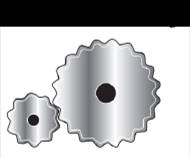Chapter 6.3, Problem 44EElementary Geometry For College St...

7th Edition
Alexander + 2 others
ISBN: 9781337614085

Solutions

Chapter
SectionElementary Geometry For College St...

7th Edition
Alexander + 2 others
ISBN: 9781337614085
Textbook Problem

If the larger gear has 30 teeth and smaller gear has 18 then the gear ratio (larger to smaller is 5:3. When the larger gear rotates through an angle of 60 ∘ , through what angle measure does the smaller gear rotate?To determine

To measure:

The angle does the smaller gear rotate.

Explanation

Consider the following condition,

“If the larger gear has 30 teeth and smaller gear has 18 then the gear ratio (larger to smaller is 5:3). When the larger gear rotates through an angle of 60

The angle between two teeth in the larger gear is,

θL=360no.of.teeth=36030=12

The angle between two teeth in the smaller gear is,

θS=360no.of.teeth=36018=20

When the larger gear rotates 60 then number of teeth has moved is given below,

no.of

Still sussing out bartleby?

Check out a sample textbook solution.

See a sample solution

The Solution to Your Study Problems

Bartleby provides explanations to thousands of textbook problems written by our experts, many with advanced degrees!

Get Started

In Exercises 7-10, find the slope of the line shown in each figure. 8.

Applied Calculus for the Managerial, Life, and Social Sciences: A Brief Approach

In Exercises 516, evaluate the given quantity. log4164

Finite Mathematics and Applied Calculus (MindTap Course List)

Find the derivative of the function. y=1+xe2x

Single Variable Calculus: Early Transcendentals

True or False: is a convergent series.

Study Guide for Stewart's Single Variable Calculus: Early Transcendentals, 8th